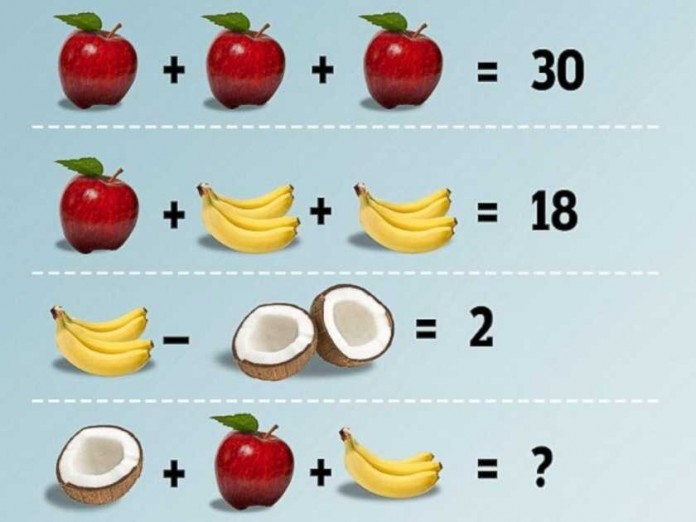# The Fruit Math Problem That is Driving Everyone Crazy

Be a Fruit Math Ninja and solve the problem!

0
24849The frustration! There has been a math riddle being shared on Facebook that is a type of fruit math problem and it’s being shared like WILDFIRE.  Some are calling it “fruit algebra” while others are just calling it the “fruit math problem” and it’s driving them nuts!

Here is the problem below, let’s see if you can figure it out first and then later I’ll walk you through the steps on one possible way to solve the problem.  You’ll need to be familiar with Algebra though.Here is the math riddle: The Fruit Math Problem. Try it by yourself and then let’s solve it together!

The crazy thing is, is that no one can agree on an answer to the problem! Haha!So let’s walk it down step by step.For simplicity, we’ll address an apple as X. So…

• X+X+X=30, or we can simply this to…
• 3X=30.  Divide both sides by 3 to get…
• X=10.We’ll notate Bananas as Y’s. Remember that we found that the apple is already 10.  So we have…

• 10 + Y + Y=18.  Subtract 10 from both sides to get…
• Y+Y=8.  Simplify the equation to get…
• 2Y=8.  Solve for Y by dividing each side by 2 to get…
• Y=4.  Therefore the bananas equal 4.We know the bananas are 4 already, and lets say the coconuts can be unknown Z.  The equation will look like:

•  4 – Z = 2.  Subtract 4 from both sides to get…
• -Z=-2. Multiply both sides by -1 to get…
•  Z=2Now many people will try to solve it like 2+10+4 = 16

HOWEVER, the coconut is a different picture!  It’s only one half the coconut and not two halves.So if we know that 2 halves of the coconuts equals 2, then 1 half equals 1.

So now we can finally solve it, right? and we get 15?

## Not so FAST!Bet you didn’t look close at the bananas! WHAT?! Because the bananas are a different image too!  Instead of seeing 4 bananas in the cluster, we only see 3 bananas (Some still argue they see 4).   Since the original cluster of 4 bananas equaled 4. That means that each banana is worth 1.  This group of 3 bananas equals 3.

So… Finally… we can solve: 1 + 10 + 3 = 14.  And the fruit math problem is finally solved.

## 14

Now for the people out there who are math majors will probably be shaking their heads right now because TECHNICALLY… you really can’t associate how a fruit image (variable) looks to it’s value.  It would really be a different variable all together.  That’s like me saying that V is half the value of X because it looks like two V’s make up the top and bottoms of the X.  BUT, that’s only if you’re saying that the fruit images are ONLY variables. Anyways, this is all in good fun and it’s more of a math riddle and math problem mixed into one than just a pure math problem alone.

## How did you do on the Fruit Math Problem?

Let us know in the comments below! Did you get it the first time, or did the images trick you? Or do you not agree?

Hope you enjoyed this math riddle!  Share this with your friends with the buttons below to see if they can solve it too!